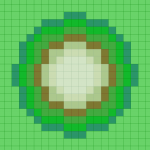## ST_DWithin on a Grid

As part of a larger inquiry, I wanted to know how `ST_DWithin()` behaved on a regular grid – that is, what is the relationship of the input radius, and the number of grid cells that could be selected in the result. The manual implies that it is an intersects relationship not a contains relationship, and in this case, the center is not a point but rather an entire grid cell.

Empirically I came up with a formula that does a good enough job of determining a maximum number of grid cells that could be selected (the logic for the inner case of a small radius is rough, but that is almost inconsequential). Here is a SQL query as executed in openJump, and a screenshot:```SELECT  st_asbinary(r.wkb_geometry)
FROM    grid150m_county r
JOIN    grid150m_county a ON
ST_DWithin( r.wkg_geometry, a.wkb_geometry, tRadius)
AND a.ogc_fid = tCellID
GROUP BY
r.wkb_geometry
```

A possible Python function to get a maximum count of grid cells:

```##-----------------------------------------------------------
import math
def get_gridcell_count( inRadius, inCellSize=150.0 ):

## for a given R in meters, get the max number of 150m grid cells
##  that could be included in the results search
## Empirically determined to be factorial-like function
## adding a new ring at each new grid cell size increment
##
##  tRadius is in meters

if inRadius < inCellSize:  return 1

tGridCellsSum = 9